# EM Analysis Using Sonnet Software

Here's a separate page analyzing the loss of microstrip on silicon, a case where substrate resistivity is not at its ideal (infinite) value.

Way back in 2007, we installed SONNET on a Dell Dimension 4700 computer (low end hardware), and waded through the first two tutorials. We like how fast it runs, and look forward to posting some examples that we will design ourselves (not just stuff from their manuals!)

### Box size

Electromagnetic analysis uses finite element math. At best it is a good approximation of how a circuit will behave, and is more accurate than linear analysis software. At worst, it is bad approximation, and a big waste of a your time because it can run very slowly. The "art" of EM analysis is knowing when you have a good approximation, and how to take shortcuts that save time but don't corrupt the analysis. So we will start with some very simple circuits that will help us (and you) check the accuracy of the analysis.

In SONNET, the "finite element" that is being analyzed zillions of times is a rectangle that you define, which they call a "box". Choose a box that is too big, and your approximation will be off. Choose a box size that is too small, and you'll be waiting a long time to analyze even the simplest structures. We'll discuss this more in Project #2 below.

### Project #1: loss of fifty-ohm line on 2-mil GaAs

We're not huge fans of reading manuals or analyzing example files, so after an hour of following the tutorial, we jumped right in and created our own circuit. In this case, it was a fifty ohm line! Not that interesting you say, unless you are curious about the loss of fifty ohm lines up at 100 GHz, on 2-mil GaAs, due to skin depth.

Without too much fuss, we built up the following geometry: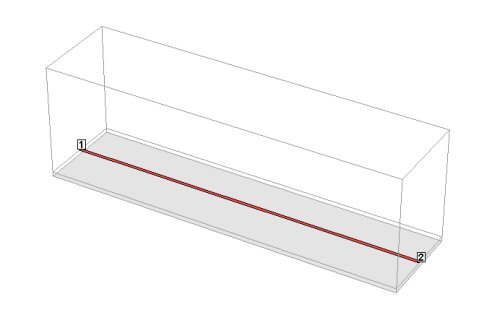We really like SONNET's cool 3D viewer, as well as the "quick start guide", which sits on screen and prods you through each step of the analysis. We created a 2 mil GaAs (ER=12.9) substrate, with a "cover" height of 50 mils. We used a metal thickness of 0.15 mils, choosing gold as the conductor (which automatically sets the conductivity to 40900000 Siemens/meter). The line width is 1.4 mils, and length is 160 mils (experience told us that 1.4 mils is close to fifty ohms). Originally we set the "box size" to 0.1 mils in both X and Y directions, but soon learned that the circuit was gonna take all day to analyze. So we switched to 1.0 mils in X and 0.2 mils in Y, and it ran in a couple of minutes. Here are the results: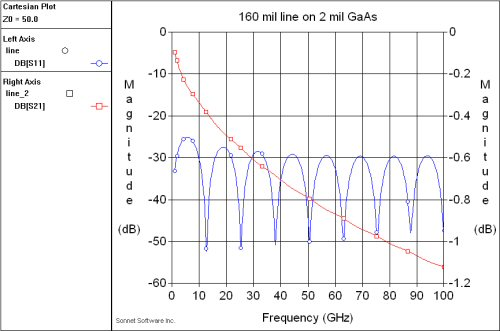Looks like our width of 1.4 mils was a good guess for fifty ohms (-30 dB match is good enough for government work!) The loss at 100 GHz is -1.12 dB. Because the circuit is well matched, we can ignore the mismatch loss, and compute the loss of this medium at 100 GHz, in dB/inch, and dB/cm:

Loss of 2-mil GaAs 50-ohm line at 100 GHz= -7 dB/inch, or -2.75 dB/cm

Why is there a loss of -0.1 dB down at DC (0 Hertz)? That's because this particular fifty ohm line has 6 milliohms/square DC sheet resistance (you can do the math for homework!), and there are a total of 133 squares of metal. This is certainly something you need to take into account if you want to pass some DC current through it.

Let's check our "skin depth rule of thumb" that says you need only five skin depths to achieve maximum conductivity. Using our skin depth calculator, we find that at 100 GHz, the skin depth is just under 10 microinches. The line above was 150 microinches... what happens if we decrease it down to 50 uin? Here's the new response: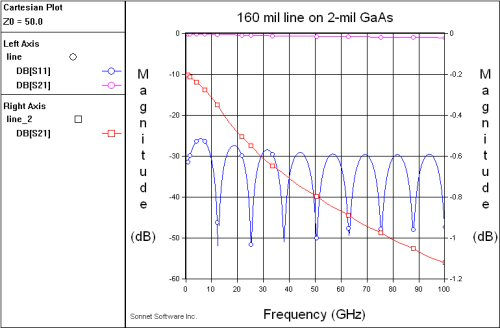The DC loss is has increased to -0.2 dB, but the loss at 100 GHz is exactly the same at 1.12 dB, so 50 uin is as good as 150 uin at this frequency. Suppose you were a cheapskate, and tried to get away with 1 skin depth at 100 GHz (10 uin). Below is the result (we had to change the right Y-axis, it went off the page!)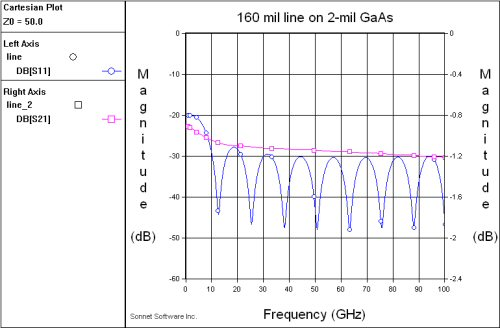The loss only increased to -1.21 dB at 100 GHz, but no microwave engineer wants to give up even a tenth of a dB if he doesn't have to. Thus SONNET EM analysis supports our rule that five skin depths is all you need!

Let's take a look at the loss that is predicted by the next iteration of our RF sheet resistance spreadsheet: for the same geometry (160 mil 50-ohm line, metal thickness 10 microinches). Looks like it is off a bit, now that we have SONNET we can figure out what is the problem!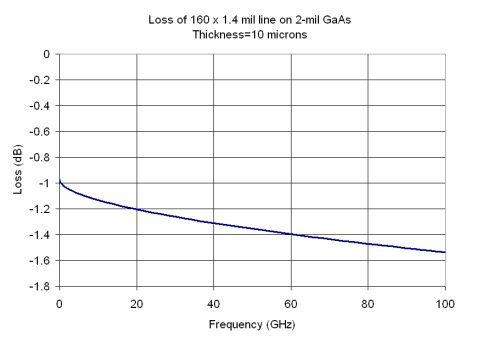### Project #2: millimeterwave terminations

While we are on 2-mil GaAs, we decided to look at "fan-style" terminations. An ideal termination acts like a simple shunt 50 ohm resistor, the center of the Smith chart. Using via holes for ground adds unwanted series reactance due to via inductance which increases with frequency. An open stub can achieve a "perfect short" over a moderate bandwidth, but no one trusts linear models (such as ADS or Eagleware) at millimeterwave frequencies to get the exact dimensions. So we used SONNET to analyze some fifty-ohm termination structures.

The first thing we had to do was come up with a SONNET metalization layer that provides nominal 50 ohms per square sheet resistance. By selecting tantalum in the "metal types" pull down, the bulk conductivity is given as 6450000 S/m. Using Excel as a scratchpad, we calculated that a metal thickness of 0.000122 mils would give us 50.0 ohms/mm (sheet resistance is (1/(conductivity*metal thickness, just make sure the units are consistent).

In a new project we created a fan stub, from the "tools", "add metalization" menu. We chose a length of ten mils, which is a quarterwave somewhere around 50 GHz. The box size initially was still set to 0.2 x 1.0 mils; the actual geometry that Sonnet analyzes is shown in the staircase outline below. The green feature is the fifty-ohm resistor, which in this case measures 1 mil x 1 mil. This analysis took 21 seconds.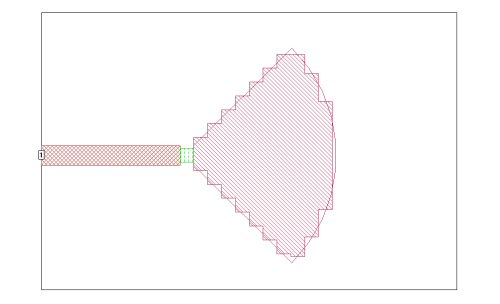After we analyzed the case shown above, we changed the box size down to 0.2 x 0.2 mils, which gives a much more accurate outline of the fan stub. This is shown in the following figure. This analysis took 51 seconds.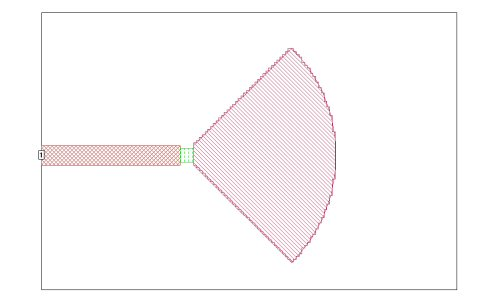We ran both cases to see what error is introduced by using the cruder approximation; the two traces below show that an error of about 2 GHz (4%) in center frequency is the result. In this case, it is apparent that the box size in the first run was too large for a "final design", but might be just fine for preliminary design work where you want speedy, if not 100% accurate answers.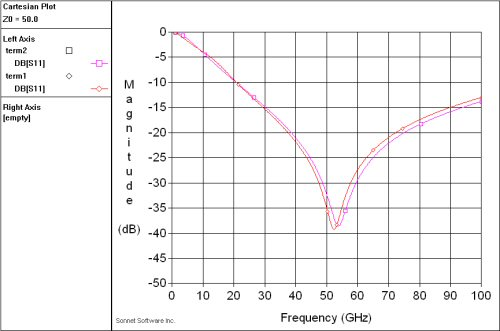By the way, what happens if we merely ground the 1x1 mil fifty-ohm resistor with a via hole? Below is the response computed by Sonnet. It looks great down at X-band, but the fan stub termination does much better at 50 GHz.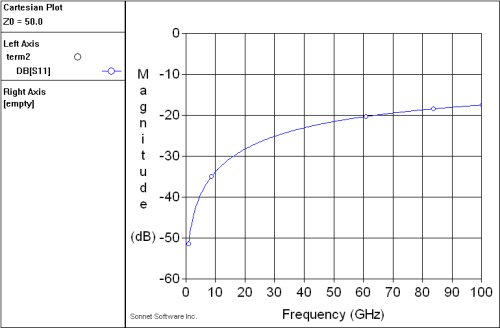Author : Unknown Editor